roman_max
☆

Russia,
2019-04-23 16:01

Posting: # 20210
Views: 3,752

## Graphing Mean PK profile [R for BE/BA]

Dear R-users,

recently I received a request from Sponsor to represent mean PK profile in a box-and-whiskers fashion with application of a mean connection line in one graph. Guess, ggplot2 can do this job, but unfortunately Im not so familiar with such sofisticated plot-making for PK profile.
Can anyone share idea (R-code?) how to do it? How a data-set can be organized for this graph?
Shuanghe
★★

Spain,
2019-04-23 18:31

@ roman_max
Posting: # 20213
Views: 3,527

## Graphing Mean PK profile

Dear roman_max,

» Can anyone share idea (R-code?) how to do it? How a data-set can be organized for this graph?

Assuming that your data file of individual concentration dat_ind contain at least the following variables: subj, treat, time, conc, you can get mean profile data with
library(dplyr) dat_mean <- dat_ind %>%   group_by(treat, time) %>%   summarise(conc = mean(conc))

Obviously, time  here should be the planned nominal time, not the actual sampling time. With ggplot you can have more or less what you asked for:

library(ggplot2) p1 <- ggplot(data = dat_ind, aes(x = time, y = conc, color = treat)) +   geom_point(aes(group = interaction(treat, time)), alpha = 0.5, shape = 1,              position = position_jitter(width = 0.1, height = 0)) +   geom_boxplot(aes(fill = treat, group = interaction(treat, time)), alpha = 0.3) +   geom_line(data = dat_mean, size = 1.3)

The main idea is the group = interaction(treat, time). Feel free to modify the rest to better suit your needs (size/shape of the points etc). position_jitter() helps to avoid point overlapping (you have to specify height = 0 otherwise the data points will not reflect the true concentration value since some randomness will be introduced along y-axis by default) and the last line will add mean profiles with slightly bold lines.

All the best,
Shuanghe
roman_max
☆

Russia,
2019-04-24 12:29

@ Shuanghe
Posting: # 20221
Views: 3,441

## Graphing Mean PK profile

Dear Shuanghe,

Today is a beautiful dayThank you very much for your help. Ive reproduced the code and get what I wanted to see. Hope, Sponsor will be in the best feelingsHelmut
★★★Vienna, Austria,
2019-04-23 22:23

@ roman_max
Posting: # 20214
Views: 3,508

## Graphing Mean PK profile

Hi roman_max,

» recently I received a request from Sponsor to represent mean PK profile in a box-and-whiskers fashion with application of a mean connection line in one graph.

The sponsor should re-consider this idea. Box-plots are nonparametric. For log-normal distributed data (which we likely have) the median is an estimate of the geometric mean. If we want to go this way, the arithmetic mean is not a good idea.

» Can anyone share idea (R-code?) how to do it? How a data-set can be organized for this graph?

An idea, yes. I borrowed mittyri’s simulation code. With real data work with the second data.frame.

C <- function(F=1, D, Vd, ka, ke, t) {   C <- F*D/Vd*(ka/(ka - ke))*(exp(-ke*t) - exp(-ka*t))   return(C) } Nsub     <- 24 D        <- 400 ka       <- 1.39 ka.omega <- 0.1 Vd       <- 1 Vd.omega <- 0.2 CL       <- 0.347 CL.omega <- 0.15 t        <- c(seq(0, 1, 0.25), seq(2,6,1), 8,10,12,16,24) ke       <- CL/Vd tmax     <- log((ka/ke)/(ka - ke)) Cmax     <- C(D=D, Vd=Vd, ka=ka, ke=ke, t=tmax) LLOQ.pct <- 2 # LLOQ = 2% of theoretical Cmax LLOQ     <- Cmax*LLOQ.pct/100 df1      <- data.frame(t=t) for (j in 1:Nsub) {   ka.sub <- ka * exp(rnorm(1, sd = sqrt(ka.omega)))   Vd.sub <- Vd * exp(rnorm(1, sd = sqrt(Vd.omega)))   CL.sub <- CL * exp(rnorm(1, sd = sqrt(CL.omega)))   df1    <- cbind(df1, C(D=D, Vd=Vd.sub, ka=ka.sub, ke=CL.sub/Vd.sub, t=t))   df1[which(df1[, j+1] < LLOQ), j+1] <- NA } names(df1)[2:(Nsub+1)] <- paste0("S.", 1:Nsub) df2 <- data.frame(t(df1[-1])) colnames(df2) <- df1[, 1] names(df2) <- t print(signif(df2, 3)) # show what we have plot(x=t, y=rep(0, length(t)), type="n", log="y", xlim=range(t),      ylim=range(df2, na.rm=TRUE), xlab="time",      ylab="concentration", las=1) for (j in seq_along(t)) {   bx <- boxplot(df2[, j], plot=FALSE)   if (bx$n > 0) bxp(bx, log="y", boxwex=0.25, at=t[j], axes=FALSE, add=TRUE) } Which gave in one run:  0 0.25 0.5 0.75 1 2 3 4 5 6 8 10 12 16 24 S.1 NA 199.0 304 353 367 295.0 198.0 128.00 82.2 52.60 21.40 8.75 NA NA NA S.2 NA 132.0 218 269 296 278.0 199.0 129.00 79.7 47.90 16.70 5.65 NA NA NA S.3 NA 82.7 121 136 141 126.0 106.0 88.30 73.5 61.30 42.50 29.50 20.50 9.87 NA S.4 NA 150.0 202 204 184 76.8 24.3 6.91 NA NA NA NA NA NA NA S.5 NA 102.0 167 206 226 216.0 160.0 110.00 72.6 47.30 19.70 8.16 NA NA NA S.6 NA 132.0 213 260 285 285.0 241.0 197.00 160.0 129.00 84.30 55.10 36.00 15.40 NA S.7 NA 96.9 157 193 213 216.0 185.0 152.00 124.0 101.00 67.20 44.50 29.50 13.00 NA S.8 NA 84.1 141 179 203 229.0 213.0 189.00 165.0 143.00 108.00 81.70 61.70 35.20 11.40 S.9 NA 183.0 283 332 349 296.0 211.0 146.00 99.5 67.90 31.50 14.60 6.80 NA NA S.10 NA 93.8 150 181 194 166.0 110.0 66.40 38.6 22.10 7.03 NA NA NA NA S.11 NA 148.0 236 284 304 261.0 176.0 109.00 65.9 39.10 13.60 NA NA NA NA S.12 NA 95.3 156 193 213 206.0 159.0 114.00 79.8 55.30 26.30 12.50 5.89 NA NA S.13 NA 69.3 120 157 182 214.0 197.0 166.00 135.0 108.00 67.40 41.80 25.90 9.91 NA S.14 NA 73.8 118 144 157 157.0 133.0 109.00 89.2 72.70 48.30 32.10 21.30 9.43 NA S.15 NA 245.0 383 453 481 419.0 304.0 213.00 147.0 101.00 48.10 22.90 10.90 NA NA S.16 NA 97.4 157 192 211 204.0 163.0 124.00 93.4 69.90 39.00 21.80 12.20 NA NA S.17 NA 71.9 119 149 167 179.0 160.0 136.00 115.0 96.50 67.90 47.80 33.60 16.60 NA S.18 NA 133.0 203 232 236 159.0 80.0 35.90 15.1 6.10 NA NA NA NA NA S.19 NA 209.0 307 340 338 226.0 127.0 68.00 36.2 19.20 5.39 NA NA NA NA S.20 NA 156.0 235 266 267 172.0 83.5 36.00 14.6 5.66 NA NA NA NA NA S.21 NA 158.0 261 325 360 355.0 274.0 196.00 136.0 92.60 42.50 19.40 8.83 NA NA S.22 NA 97.0 163 206 233 248.0 210.0 165.00 127.0 96.30 54.90 31.20 17.70 5.69 NA S.23 NA 114.0 182 220 240 240.0 208.0 175.00 147.0 123.00 86.70 60.90 42.80 21.20 5.17 S.24 NA 121.0 195 238 261 260.0 216.0 173.00 137.0 109.00 67.90 42.40 26.50 10.40 NA The bad thing is that you have to use very narrow boxes in order to avoid overlaps (boxwex=0.25).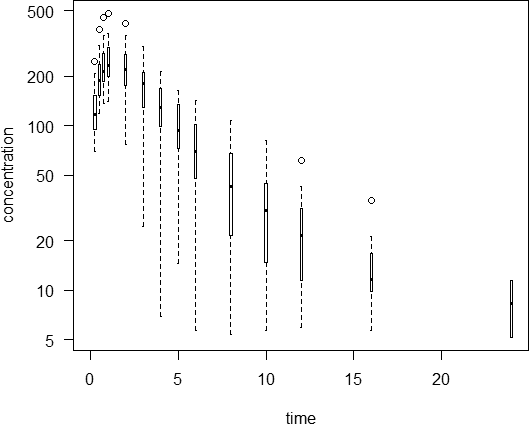In my “bible” that’s called a high ink-to-information ratio* which is bad style. One option would be to draw a thick line instead of the box, a thin line for the whiskers, and smaller points for the outliers, e.g., plot(x=t, y=rep(0, length(t)), type="n", log="y", xlim=range(t), ylim=range(df2, na.rm=TRUE), xlab="time", ylab="concentration", las=1) for (j in seq_along(t)) { bx <- boxplot(df2[, j], plot=FALSE) if (bx$n > 0) {     lines(rep(t[j], 2), c(bx$stats[1, 1], bx$stats[5, 1]))     lines(rep(t[j], 2), c(bx$stats[2, 1], bx$stats[4, 1]), lwd=3, col="gray50")     points(t[j], bx$stats[3, 1], pch=3, cex=0.6) points(rep(t[j], length(bx$out)), bx$out, pch=1, cex=0.5) } }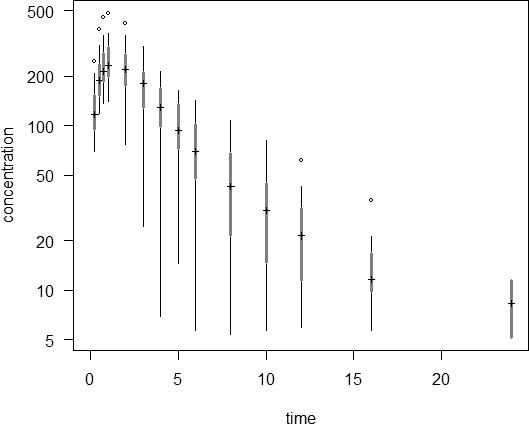Add a line connecting the medians and you are doomed. • Tufte ER. The Visual Display of Quantitative Information. 2nd ed. Cheshire: Graphics Press; 2001. Edit: Hey, Shuanghe – you were much faster! Cheers, Helmut SchützThe quality of responses received is directly proportional to the quality of the question asked. 🚮 Science Quotes nobody nothing 2019-04-24 11:23 @ Helmut Posting: # 20215 Views: 3,460 ## Graphing Mean PK profile ...if you want to plot T and R in one graph I would suggest "stacked" or "pseudo-3D" view, by adding/subtracting a few minutes to the actual time for T/R... Kindest regards, nobody Helmut ★★★Vienna, Austria, 2019-04-24 11:49 @ nobody Posting: # 20217 Views: 3,478 ## Graphing Mean PK profile Hi nobody, » ...if you want to plot T and R in one graph I would suggest "stacked" or "pseudo-3D" view, by adding/subtracting a few minutes to the actual time for T/R... I make it sometimes upon sponsor’s demand. Personally I think that such a plot is too “crowded”.Cheers, Helmut SchützThe quality of responses received is directly proportional to the quality of the question asked. 🚮 Science Quotes nobody nothing 2019-04-24 11:53 @ Helmut Posting: # 20218 Views: 3,439 ## Graphing Mean PK profile ..add some more minutes and keep the symbols smallerKindest regards, nobody Helmut ★★★Vienna, Austria, 2019-04-24 12:32 @ nobody Posting: # 20222 Views: 3,446 ## Graphing Mean PK profile Hi nobody, » ..add some more minutes and keep the symbols smallerLike this? ±10 instead of ±5 and symbols –40%: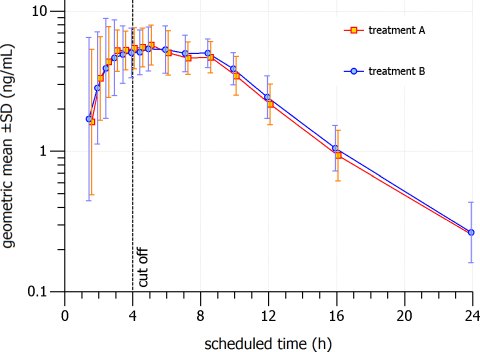Cheers, Helmut SchützThe quality of responses received is directly proportional to the quality of the question asked. 🚮 Science Quotes nobody nothing 2019-04-24 12:49 @ Helmut Posting: # 20224 Views: 3,421 ## Graphing Mean PK profile I would prefer the blue ones on the right side, but otherwise: Yepp... Kindest regards, nobody roman_max ☆ Russia, 2019-04-24 12:36 @ Helmut Posting: # 20223 Views: 3,419 ## Graphing Mean PK profile Dear Helmut, thank you very much for the contribution to my knowledgeIndeed, very useful. » The sponsor should re-consider this idea. Box-plots are nonparametric. For log-normal distributed data (which we likely have) the median is an estimate of the geometric mean. If we want to go this way, the arithmetic mean is not a good idea. Agree, but if it is easier for Sponsor to view and "understand" data, no problem. Helmut ★★★Vienna, Austria, 2019-04-24 13:50 @ roman_max Posting: # 20225 Views: 3,428 ## Graphing Mean PK profile Hi roman_max, » » The sponsor should re-consider this idea. Box-plots are nonparametric. For log-normal distributed data (which we likely have) the median is an estimate of the geometric mean. If we want to go this way, the arithmetic mean is not a good idea. » » Agree, but if it is easier for Sponsor to view and "understand" data, no problem. Any kind of plot is problematic. Given, some guidelines require them (e.g., the Canadian guidance). We should be aware that such a plot gives just an impression and is not related with the assessment of BE. I once received a deficiency letter asking for a clarification why in plots of geometric mean profiles the highest concentrations and their time points didn’t agree with the reported Cmax/tmax. Well, C at any given time point has nothing to do with the individual Cmax-values and their geometric mean. Oh, dear! There’s another obstacle. How to deal with BQLs? If you set them to NA (in R) or keep them as a character-code (Phoenix/WinNonlin) you open Pandora’s box. Let’s have a look at the 16 h time point of my example: loc.stat <- function(x, type, na.rm) { non.numerics <- which(is.na(suppressWarnings(as.numeric(x)))) x[non.numerics] <- NA x <- as.numeric(x) switch(type, arith.mean = round(mean(x, na.rm=na.rm), 2), median = round(median(x, na.rm=na.rm), 2), geom.mean = round(exp(mean(log(x), na.rm=na.rm)), 2), harm.mean = round(length(x)/sum(1/x, na.rm=na.rm), 2)) } C <- c(rep("BQL", 2), 9.87, rep("BQL", 2), 15.4, 13.0, 35.2, rep("BQL", 4), 9.91, 9.43, rep("BQL", 2), 16.6, rep("BQL", 4), 5.69, 21.2, 10.4) df <- data.frame(statistic=c(rep("arith.mean", 2), rep("median", 2), rep("geom.mean", 2), rep("harm.mean", 2)), na.rm=rep(c(FALSE, TRUE), 4), location=NA, stringsAsFactors=FALSE) for (j in 1:nrow(df)) { df$location[j] <- loc.stat(C, df$statistic[j], df$na.rm[j]) } print(df, row.names=FALSE)   statistic na.rm location  arith.mean FALSE       NA  arith.mean  TRUE    14.67      median FALSE       NA      median  TRUE    11.70   geom.mean FALSE       NA   geom.mean  TRUE    12.98   harm.mean FALSE       NA   harm.mean  TRUE    27.98 # not meaningful, only for completeness

Some people set all BQLs to zero in order to calculate the arithmetic mean. Others set the first BQL after tmax to LLOQ/2, and, and, and… There will always be a bias.

Cheers,
Helmut SchützThe quality of responses received is directly proportional to the quality of the question asked. 🚮
Science Quotes
nobody
nothing

2019-04-24 14:15

@ Helmut
Posting: # 20226
Views: 3,412

## Graphing Mean PK profile

» Some people set all BQLs to zero in order to calculate the arithmetic mean. Others set the first BQL after tmax to LLOQ/2, and, and, and… There will always be a bias.

How would you otherwise calculate the geo. mean profile?

And AUCtlast is a mess with different tlast for T and R and and and. Statistics is always some kind of abstraction. Do a spaghetti plot for T and R and individual T+R for each subject and you might get completely different "ideas" / insights...

Kindest regards, nobody
Helmut
★★★Vienna, Austria,
2019-04-24 14:41

@ nobody
Posting: # 20227
Views: 3,535

## Graphing Mean PK profile

Hi nobody,

» » Some people set all BQLs to zero in order to calculate the arithmetic mean. Others set the first BQL after tmax to LLOQ/2, and, and, and… There will always be a bias.
»
» How would you otherwise calculate the geo. mean profile?

Tons of rules. Mine: If at any given time point ≥⅔ of concentrations are ≥LLOQ I calculate the geometric mean of those (i.e., exclude the BQLs). If less, I don’t calculate the geometric mean at all. Luckily in my studies the LLOQ was always low enough.Had a look at the Canadian guidance and the example given in the appendix. HC calculates the arithmetic (!) mean whilst treating BQLs as zero (Table A1-B/C, p.23/24). If a mean is <LLOQ, it is not shown in the plot (Figure 1, p.31).

Played a bit with the example (after correcting the typo 3723 → 37.23 which sits happily in the table for 25 years). R-code at the end.
                             method  0 0.33  0.67     1   1.5     2     3     4     6     8   12  16          arithm.mean (BQL excluded)  0  NaN 60.44 73.28 82.85 70.94 49.62 29.09 17.19 10.38 6.56 NaN              arithm.mean (BQL => 0)  0  0.0 45.33 73.28 82.85 70.94 49.62 29.09 17.19  6.49 1.64 0.0 arithm.mean (BQL => 0, NA if <LLOQ) NA   NA 45.33 73.28 82.85 70.94 49.62 29.09 17.19  6.49   NA  NA         arithm.mean (BQL => LLOQ/2)  0  2.5 45.95 73.28 82.85 70.94 49.62 29.09 17.19  7.42 3.52 2.5            geom.mean (BQL excluded)  0  NaN 39.58 57.55 71.98 61.44 45.24 26.08 14.98  9.74 6.51 NaN           geom.mean (BQL => LLOQ/2)  0  2.5 19.84 57.55 71.98 61.44 45.24 26.08 14.98  5.85 3.18 2.5 geom.mean (if at least 2/3 >= LLOQ)  0   NA 39.58 57.55 71.98 61.44 45.24 26.08 14.98    NA   NA  NA               median (BQL excluded)  0   NA 40.97 54.39 67.57 63.05 44.69 26.46 16.86  9.19 6.62  NA              median (BQL => LLOQ/2)  0  2.5 33.24 54.39 67.57 63.05 44.69 26.46 16.86  6.84 2.50 2.5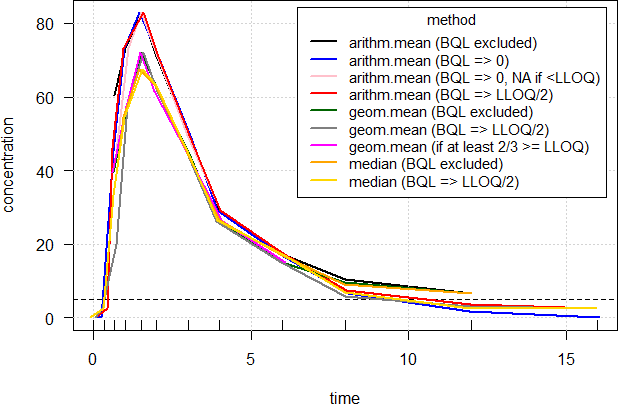Added some jitter to separate the lines.
Now what? I think everything (except arithmetic means) is OK. Coming up with sumfink which is <LLOQ? Hhm. As soon as we have a single value <LLOQ it might be that the estimate is <LLOQ as well (if all others are LLOQ). But how much? No idea.

Will submit a study to HC soon. Will report back how they like my approach.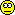» And AUCtlast is a mess with different tlast for T and R and and and.

Can’t agree more.

» Do a spaghetti plot for T and R and individual T+R for each subject and you might get completely different "ideas" / insights...

Yes and yes. Henning Blume always apologized when presenting (arithmetic) mean plots. I’m pissed when I get a report without spaghetti plots.

loc.stat <- function(x, type, na.rm) {   non.numerics    <- which(is.na(suppressWarnings(as.numeric(x))))   x[non.numerics] <- NA   x <- as.numeric(x)   switch(type,          arith.mean = round(mean(x, na.rm=na.rm), 2),          median     = round(median(x, na.rm=na.rm), 2),          geom.mean  = round(exp(mean(log(x), na.rm=na.rm)), 2)) } t <- c(0, 0.33, 0.67, 1, 1.5, 2, 3, 4, 6, 8, 12, 16) LLOQ <- 5 df <- data.frame(ID=c("A", "B", "C", "E", "F", "G", "H", "I",                       "K", "L", "M", "N", "O", "P", "Q", "R"),                  t.1=rep(0, 16), t.2=rep("BQL", 16),                  t.3=c(116.40,  88.45,  "BQL",  37.23,  29.25,   6.89, 113.50, 181.90,                         42.71,  14.29,   8.21,  47.20,  "BQL",  39.23,  "BQL",  "BQL"),                  t.4=c(124.60, 121.40,  95.57,  37.26,  62.88,  50.04, 218.70, 135.80,                         58.75,  21.32,  48.87,  34.90,  20.35,  86.29,  30.86,  24.84),                  t.5=c(126.20, 206.90, 122.80,  35.90,  64.26,  55.27, 125.80,  96.51,                         59.68,  24.32,  57.05,  34.90,  70.88,  97.46,  88.38,  59.27),                  t.6=c(107.60, 179.00, 103.20,  28.87,  84.67,  51.68,  69.77,  90.50,                         54.37,  25.56,  56.32,  24.19,  70.60,  52.26,  37.67,  98.82),                  t.7=c( 45.65,  84.53, 101.70,  28.48,  45.21,  38.58,  45.03,  62.58,                         44.35,  25.51,  42.08,  20.11,  70.38,  40.53,  29.28,  69.98),                  t.8=c( 33.22,  40.02,  57.65,  25.10,  25.05,  26.19,  32.78,  30.43,                         22.94,  10.49,  24.79,   8.08,  40.51,  26.74,  14.99,  46.50),                  t.9=c( 16.11,  38.01,  23.85,  24.91,  17.18,   7.79,  18.55,  18.50,                         11.58,   5.49,  16.54,   7.27,  26.93,  12.54,   6.38,  23.46),                 t.10=c( 12.60,  15.12,  14.59,   6.72,   8.47,  "BQL",   5.42,  "BQL",                          6.95,  "BQL",  15.81,  "BQL",   8.20,  "BQL",  "BQL",   9.91),                 t.11=c( "BQL",   5.39,   6.29,  "BQL",  "BQL",  "BQL",  "BQL",  "BQL",                         "BQL",  "BQL",   7.60,  "BQL",  "BQL",  "BQL",  "BQL",   6.96),                 t.12=rep("BQL", 16), stringsAsFactors=FALSE) loc <- data.frame(method=c("arithm.mean (BQL excluded)",                            "arithm.mean (BQL => 0)",                            "arithm.mean (BQL => 0, NA if <LLOQ)",                            "arithm.mean (BQL => LLOQ/2)",                            "geom.mean (BQL excluded)",                            "geom.mean (BQL => LLOQ/2)",                            "geom.mean (if at least 2/3 >= LLOQ)",                            "median (BQL excluded)",                            "median (BQL => LLOQ/2)"),                   t.1=NA, t.2=NA, t.3=NA, t.4=NA, t.5=NA, t.6=NA,                   t.7=NA, t.8=NA, t.9=NA, t.10=NA, t.11=NA, t.12=NA,                   stringsAsFactors=FALSE) names(df)[2:13] <- names(loc)[2:13] <- t for (j in 2:ncol(df)) {    x <- df[, j]    loc[1, j] <- loc.stat(x, "arith.mean", TRUE)    y <- x    y[which(x == "BQL")] <- 0    loc[2, j] <- loc.stat(y, "arith.mean", FALSE)    ifelse(loc[2, j] >= LLOQ, loc[3, j] <- loc[2, j], loc[3, j] <- NA)    y <- x    y[which(x == "BQL")] <- LLOQ/2    loc[4, j] <- loc.stat(y, "arith.mean", FALSE)    loc[5, j] <- loc.stat(x, "geom.mean", TRUE)    loc[6, j] <- loc.stat(y, "geom.mean", FALSE)    if (length(which(x != "BQL")) >= nrow(df)*2/3) {       loc[7, j] <- loc[4, j]    } else {       loc[7, j] <- NA    }    loc[8, j] <- loc.stat(x, "median", TRUE)    loc[9, j] <- loc.stat(y, "median", FALSE) } clr <- c("black", "blue", "pink", "red", "darkgreen",          "gray50", "magenta", "orange", "gold") print(df, row.names=FALSE);print(loc, row.names=FALSE) plot(t, loc[1, 2:ncol(df)], type="l", las=1, col=clr, lwd=2,      ylim=c(0, max(loc[, 2:ncol(df)], na.rm=TRUE)),      xlab="time", ylab="concentration") abline(h=LLOQ, lty=2) grid(); rug(t) for (j in 2:9) {   lines(jitter(t, factor=1.5), loc[j, 2:ncol(df)], col=clr[j], lwd=2) } legend("topright", bg="white", inset=0.02, title="method",        legend=loc[, 1], lwd=2, col=clr, cex=0.9)

Cheers,
Helmut SchützThe quality of responses received is directly proportional to the quality of the question asked. 🚮
Science Quotes
Ohlbe
★★★

France,
2019-04-25 12:47

@ Helmut
Posting: # 20228
Views: 3,297

## Pasta

Dear Helmut,

» I’m pissed when I get a report without spaghetti plots.

And you complain that overlaying two plots of means is too crowdedI find spaghetti plots rather difficult to read with more than 30 subjects, or even less depending on what they look like...

Regards
Ohlbe
nobody
nothing

2019-04-25 14:36

@ Ohlbe
Posting: # 20230
Views: 3,267

## Pasta

I don't read 'em, I have a quick look, "see" the variability/"outliers" and job done.

Totally correct that more than 36 is hard to see anything, but if all profiles are pretty close that's in important info, too, imho. 'Kindest regards, nobody
Helmut
★★★Vienna, Austria,
2019-04-25 14:39

@ Ohlbe
Posting: # 20231
Views: 3,305

## Spaghetti Viennese

Dear Ohlbe,

» » I’m pissed when I get a report without spaghetti plots.
»
» And you complain that overlaying two plots of means is too crowdedNope. Overlaying two plots ±SD. I’m fine with one plot of both T and R plus two showing the treatments separately (geom. mean ±SD).

» I find spaghetti plots rather difficult to read with more than 30 subjects, or even less depending on what they look like...

You get used to it.Our brains are great in pattern-recognition. Two examples (amoxicillin 1 g tablets, different tests but same reference in both studies) of Blume/Mutschler*

Study  metric    90% CI  ────────────────────────   1     AUC    92 – 111%         Cmax   87 – 109%   2     AUC    82 –  99%         Cmax   75 –  99%

Both studies passed (limits for AUC 80–125% and for Cmax 70–143%).
@ Mittyri: Good ol’ days!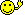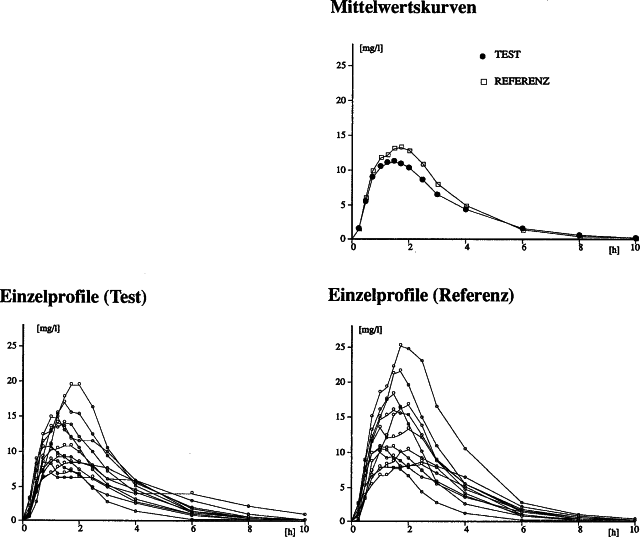I would say:
• Higher concentrations in the second study don’t bother me. Different subjects. The analytical method in the second one was 5times more sensitive and slightly more precise. The former explains why in the first study profiles in some subjects could not be measured up to the last sampling time.
• In the second study both treatments were absorbed slightly faster than in the first one.
• Now it gets interesting. In the mean curves we see that in the second study the profiles close to Cmax are more flat (independent from the formulation). However, individual profiles (yep, the pasta) of the reference in both studies were similar whereas the ones of the tests not. I prefer the second test.
This loose leaf edition (~2,000 pages in 4 volumes, 1989–1996) was prepared for the ABDA („Bundesvereinigung Deutscher Apotherverbände” – Federal Union of German Associations of Pharmacists) to provide pharmacists a means to decide which generic to chose. Already in 1992 ⅓ of all German pharmacists completed a training on BE provided by the ZL (“Zentrallaboratorium Deutscher Apotheker” – Central Laboratory of German Pharmacists). Compare that to the FDA’s Orange Book…

• Blume H, Mutschler E, editors. Bioäquivalenz. Qualitätsbewertung wirkstoffgleicher Fertigarzneimittel. Frankurt/Main: Govi-Verlag; 6. Ergänzungslieferung 1996.

Cheers,
Helmut SchützThe quality of responses received is directly proportional to the quality of the question asked. 🚮
Science Quotes
nobody
nothing

2019-04-25 15:08

@ Helmut
Posting: # 20232
Views: 3,253

## Spaghetti Viennese

»
• Blume H, Mutschler E, editors. Bioäquivalenz. Qualitätsbewertung wirkstoffgleicher Fertigarzneimittel. Frankurt/Main: Govi-Verlag; 6. Ergänzungslieferung 1996.

WOW, somebody bought that!Kindest regards, nobody
Helmut
★★★Vienna, Austria,
2019-04-25 15:32

@ nobody
Posting: # 20233
Views: 3,246

## OT: Blume/Mutschler

Hi nobody,

» WOW, somebody bought that!Though I don’t use it any more I still like the idea behind. At a conference in Toronto 1992 I showed the first file to guys (no gals at that time) of the FDA and told the story that ⅓ of German pharmacists are already trained on BE. Jaws dropped.

As an ol’ salt you know it but for the others: The ZL sent polite letters to manufacturers asking for information (the original evaluation which lead to the approval as well as study data allowing recalculation). Observations:
• Some companies refused. Consequently in the collection there was an empty page stating just the name of the product and that the company didn’t provide any information. Very bad idea.
• Comparing different products you could see that the same study was used numerous times. Hey, the file was sold.
• Sometimes you see a lot of rotten pasta and the one “perfect” study. At that time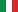was what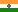is now.
• Sometimes the reported CI didn’t match the calculation of the ZL.

Cheers,
Helmut SchützThe quality of responses received is directly proportional to the quality of the question asked. 🚮
Science Quotes
nobody
nothing

2019-04-25 15:43
(edited by nobody on 2019-04-25 17:11)

@ Helmut
Posting: # 20234
Views: 3,236

## OT: Blume/Mutschler

Yepp, I know the story around that in detailIt was a move to couple science with politics (health care, pharmacists positioning as the "medicines expert"). Unfortunately this didn't work out, nowadays the health insurance buys the cheapest and decides which generic the patient gets. It's a shame...

RE: Replies from manufacturers. Not that different from today. In EU you still have only patchy Ass Reps from EMA, compared to the data released by FDA on products after MA granted.

Selling BE-studies+product was a fine business model for some time, some guys got rich and big with that. Quality of data has been an issue as long as there is any data around....Kindest regards, nobody
d_labes
★★★

Berlin, Germany,
2019-04-25 19:59

@ Helmut
Posting: # 20236
Views: 3,173

## A lie is a lie is a lie ...

Dear All,
especially the contributors to this thread.

I have a simple opinion about that mean PK profile graphs:
Whatever sophisticated group statistics underlying such mean profile you choose, whatever sophistic rules regarding missings and / or values below LLOQ, whatever sophistic graphical effects
you choose - you end with a statistical lie.
A lie
is a lie
is a lie ...

Thus my recommendation: Don't invest too many Gehirnschmalz (brain power).
Try to go without them.
And since that unfortunately doesn't function in many cases, as I know of course, choose a simple effortless solution and go with that. The Blume/Mutschler graphs shown in Helmuts post point in the correct direction.
I only had some seldom requests from some smart alecs to change the graphs. If this happens it is early enough to invest some grey matter.

Regards,

DetlewIng. Helmut Schütz